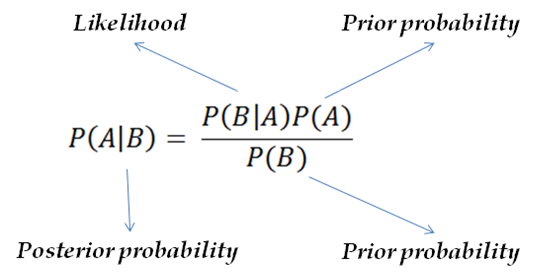Please visit, subscribe and share 10 Minutes Lectures in Computer Science

## Bayes Theorem

Bayes’ theorem is a way for finding conditional probability. Conditional probability is a probability to be found when we know about certain other probabilities (usually related).
In other words, a conditional probability could be stated as follows;
Conditional probability is about finding the probability of hypothesis H given an evidence E. That is, how often the hypothesis is true if the evidence is true?
The following tasks are some of the examples for conditional probabilities;
 Task What is to be found? (Hypothesis) What is known/given? (Evidence) What is the probability of raining if it is cloudy? Probability of rain It is cloudy What is the probability of fire if there is smoke? Probability of fire There is smoke What is the probability of the flight arriving at right time if it is a bad weather? Probability of arrival of flight at right time It is bad weather

Bayes’ theorem is a formula used to calculate conditional probability. If A and B are two events, Bayes’ theorem finds the conditional probability P(A|B) by relating another conditional probability P(B|A) with prior probabilities P(A) and P(B) as follows;The above given Bayes’ formula tells us the following;
 Bayes’ theorem finds how frequently A can happen if B happened, written as P(A|B) which is Posterior probability If we know ·         how frequently B can happen if A happened, written as P(B|A) which is Likelihood ·         how frequently A can happen on its own, written as P(A) which is Prior probability ·         how frequently B can happen on its own, written as P(B) which is Prior probability
Example:
Given the following;
·        It may be hot and dry during the month April in India. Hence, the possibility for rain is less, say 3%. [P(Rain) = 0.03]
·        Sometimes, it may be cloudy during this month (say 12%) due to moisture. [P(Cloudy) = 0.12]
·        60% of times cloudy climate causes rain during April. [P(Cloudy|Rain) = 0.6]
Find the probability of rain during the month of April if it is cloudy;
P(Rain | Cloudy) = [P(Rain) * P(Cloudy|Rain)] / P(Cloudy)
= [0.03 * 0.6] / 0.12
= 0.15 = 15%
Conclusion: 15% is the probability of rain if it is cloudy there.

***********